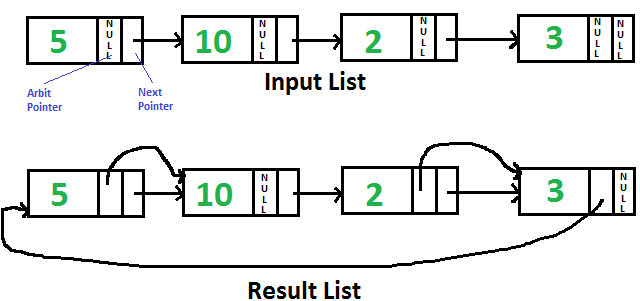# Java Program For Pointing To Next Higher Value Node In A Linked List With An Arbitrary Pointer

• Last Updated : 27 Dec, 2021

Given singly linked list with every node having an additional “arbitrary” pointer that currently points to NULL. Need to make the “arbitrary” pointer point to the next higher value node.We strongly recommend to minimize your browser and try this yourself first

A Simple Solution is to traverse all nodes one by one, for every node, find the node which has the next greater value of the current node and change the next pointer. Time Complexity of this solution is O(n2).

An Efficient Solution works in O(nLogn) time. The idea is to use Merge Sort for linked list
1) Traverse input list and copy next pointer to arbit pointer for every node.
2) Do Merge Sort for the linked list formed by arbit pointers.

Below is the implementation of the above idea. All of the merger sort functions are taken from here. The taken functions are modified here so that they work on arbit pointers instead of next pointers.

## C++

 ``` `````` `````` ``` `// Java program to populate arbit pointers  ` `// to next higher value using merge sort ` `class` `LinkedList ` `{ ` `    ``static` `Node head; ` ` `  `    ``// Link list node  ` `    ``static` `class` `Node ` `    ``{ ` `        ``int` `data; ` `        ``Node next, arbit; ` ` `  `        ``Node(``int` `data)  ` `        ``{ ` `            ``this``.data = data; ` `            ``next = null; ` `            ``arbit = null; ` `        ``} ` `    ``} ` ` `  `    ``// Utility function to print result  ` `    ``// linked list ` `    ``void` `printList(Node node, Node anode) ` `    ``{ ` `        ``System.out.println( ` `        ``"Traversal using Next Pointer"``); ` `        ``while` `(node != null) ` `        ``{ ` `            ``System.out.print(node.data + ``" "``); ` `            ``node = node.next; ` `        ``} ` ` `  `        ``System.out.println( ` `        ``"Traversal using Arbit Pointer"``); ` `        ``while` `(anode != null) ` `        ``{ ` `            ``System.out.print( ` `            ``anode.data + ``" "``); ` `            ``anode = anode.arbit; ` `        ``} ` `    ``} ` ` `  `    ``// This function populates arbit pointer  ` `    ``// in every node to the next higher value.  ` `    ``// And returns pointer to the node with  ` `    ``// minimum value ` `    ``private` `Node populateArbit(Node start) ` `    ``{ ` `        ``Node temp = start; ` ` `  `        ``// Copy next pointers to arbit  ` `        ``// pointers ` `        ``while` `(temp != null) ` `        ``{ ` `            ``temp.arbit = temp.next; ` `            ``temp = temp.next; ` `        ``} ` ` `  `        ``// Do merge sort for arbitrary pointers  ` `        ``// and return head of arbitrary pointer  ` `        ``// linked list ` `        ``return` `MergeSort(start); ` `    ``} ` ` `  `    ``/* Sorts the linked list formed by  ` `       ``arbit pointers (does not change ` `       ``next pointer or data) */` `    ``private` `Node MergeSort(Node start) ` `    ``{         ` `        ``// Base case -- length  ` `        ``// 0 or 1  ` `        ``if` `(start == null ||  ` `            ``start.arbit == null) ` `        ``{ ` `            ``return` `start; ` `        ``} ` ` `  `        ``/* Split head into 'middle' and  ` `           ``'nextofmiddle' sublists */` `        ``Node middle = getMiddle(start); ` `        ``Node nextofmiddle = middle.arbit; ` ` `  `        ``middle.arbit = null; ` ` `  `        ``// Recursively sort the sublists  ` `        ``Node left = MergeSort(start); ` `        ``Node right = MergeSort(nextofmiddle); ` ` `  `        ``/* answer = merge the two sorted  ` `           ``lists together */` `        ``Node sortedlist = SortedMerge(left, right); ` ` `  `        ``return` `sortedlist; ` `    ``} ` ` `  `    ``// Utility function to get the middle  ` `    ``// of the linked list ` `    ``private` `Node getMiddle(Node source) ` `    ``{ ` `        ``// Base case ` `        ``if` `(source == null) ` `            ``return` `source; ` `        ``Node fastptr = source.arbit; ` `        ``Node slowptr = source; ` ` `  `        ``// Move fastptr by two and slow  ` `        ``// ptr by one. Finally slowptr  ` `        ``// will point to middle node ` `        ``while` `(fastptr != null) ` `        ``{ ` `            ``fastptr = fastptr.arbit; ` `            ``if` `(fastptr != null) ` `            ``{ ` `                ``slowptr = slowptr.arbit; ` `                ``fastptr = fastptr.arbit; ` `            ``} ` `        ``} ` `        ``return` `slowptr; ` `    ``} ` ` `  `    ``private` `Node SortedMerge(Node a,  ` `                             ``Node b) ` `    ``{ ` `        ``Node result = null; ` ` `  `        ``// Base cases  ` `        ``if` `(a == null) ` `            ``return` `b; ` `        ``else` `if` `(b == null) ` `            ``return` `a; ` ` `  `        ``// Pick either a or b, and recur  ` `        ``if` `(a.data <= b.data) ` `        ``{ ` `            ``result = a; ` `            ``result.arbit =  ` `            ``SortedMerge(a.arbit, b); ` `        ``} ` `        ``else` `        ``{ ` `            ``result = b; ` `            ``result.arbit = SortedMerge(a, b.arbit); ` `        ``} ` ` `  `        ``return` `result; ` `    ``} ` ` `  `    ``// Driver code ` `    ``public` `static` `void` `main(String[] args) ` `    ``{ ` `        ``LinkedList list = ``new` `LinkedList(); ` ` `  `        ``/* Let us create the list shown ` `           ``above */` `        ``list.head = ``new` `Node(5); ` `        ``list.head.next = ``new` `Node(10); ` `        ``list.head.next.next = ``new` `Node(2); ` `        ``list.head.next.next.next = ``new` `Node(3); ` ` `  `        ``/* Sort the above created Linked List */` `        ``Node ahead = list.populateArbit(head); ` ` `  `        ``System.out.println(``"Result Linked List is:"``); ` `        ``list.printList(head, ahead); ` `    ``} ` `} ` `// This code is contributed by shubham96301     `

Output:

```Result Linked List is:
Traversal using Next Pointer
5, 10, 2, 3,
Traversal using Arbit Pointer
2, 3, 5, 10,```

Please refer complete article on Point to next higher value node in a linked list with an arbitrary pointer for more details!

My Personal Notes arrow_drop_up
Recommended Articles
Page :